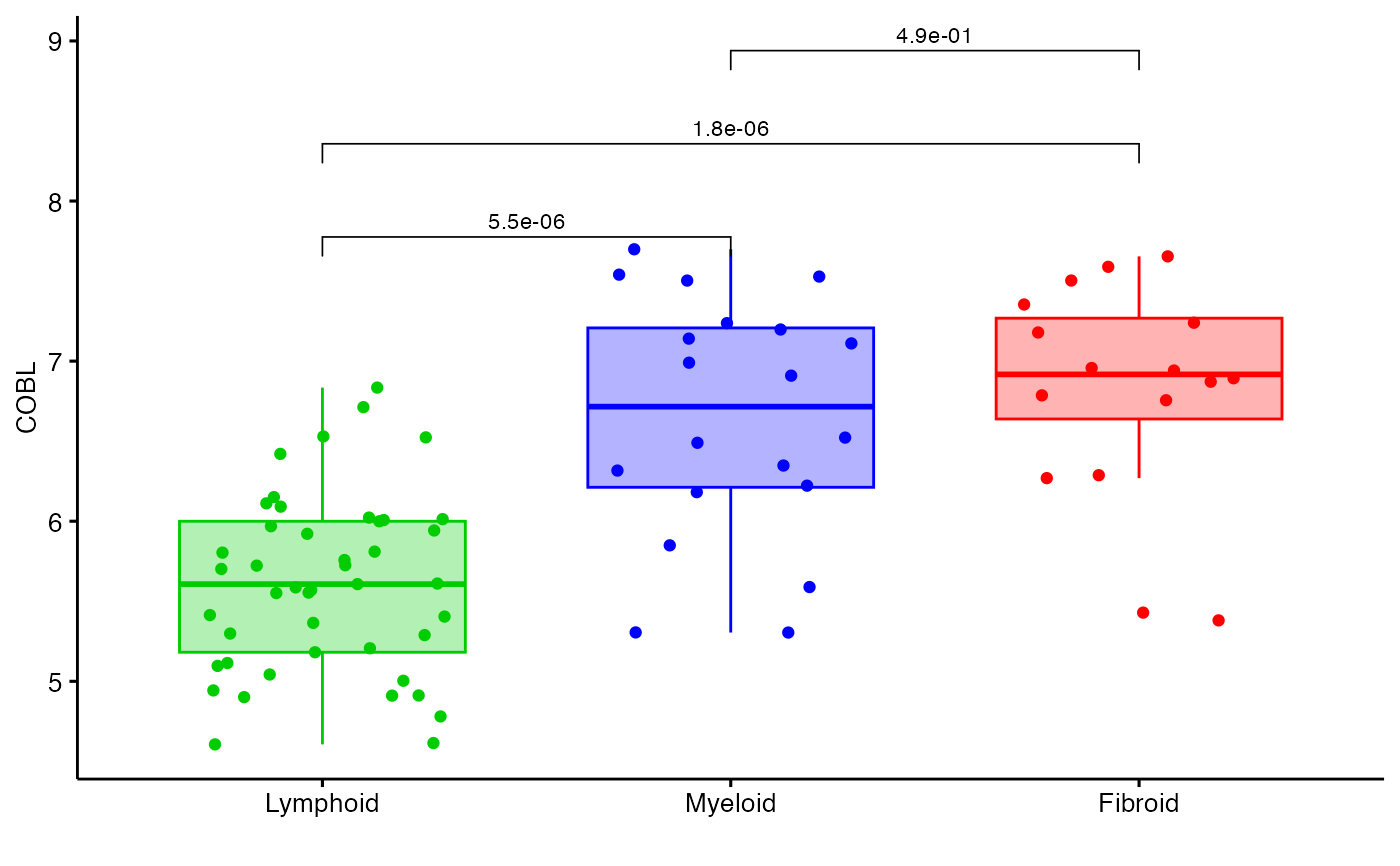Plots the expression of a specific row in expression to compare the three groups in a boxplot using either ggplot or plotly.

boxplot_trio(
polar,
value,
box_colours = c("green3", "blue", "red"),
test = "polar_pvalue",
levels_order = NULL,
my_comparisons = NULL,
text_size = 10,
stat_colour = "black",
stat_size = 3,
step_increase = 0.05,
plot_method = "ggplot",
...
)

## Arguments

polar A 'volc3d' object including expression data from groups of interest. Created by polar_coords. The column name or number in polar@data to be analysed The fill colours for each box assigned in order of levels_order. Default = c('green3', 'blue', 'red') ). The statistical test used to compare expression. Allowed values include: polar_pvalue (default) and 'polar_padj' for the pvalues and adjusted pvalues in the polar object. polar_multi_pvalue and polar_multi_padj for the pvalues and adjusted pvalues across all groups using the polar@multi_group_test  columns. t.test (parametric) and wilcox.test (non-parametric). Perform comparison between groups of samples. anova (parametric) and kruskal.test (non-parametric). Perform one-way ANOVA test comparing multiple groups. A character vector stating the contrast groups to be plotted, in order. If NULL this defaults to the levels in polar@outcome. A list of contrasts to pass to stat_compare_means. If NULL (default) all contrast pvalues are calculated and plotted. The font size of text (default = 10) Colour to print statistics (default="black"). The font size of statistical parameter (default = 3). The distance between statistics on the y-axis (default = 0.05). Whether to use 'plotly' or 'ggplot'. Default is 'ggplot' Other parameters for stat_compare_means

## Value

Returns a boxplot featuring the differential expression between groups in comparison with annotated pvalues.

Lewis, Myles J., et al. (2019). Molecular portraits of early rheumatoid arthritis identify clinical and treatment response phenotypes. Cell reports, 28:9

## Examples

data(example_data)
syn_polar <- polar_coords(outcome = syn_example_meta\$Pathotype,
data = t(syn_example_rld))

boxplot_trio(syn_polar, value = "COBL", plot_method="plotly")
boxplot_trio(syn_polar, value = "COBL")